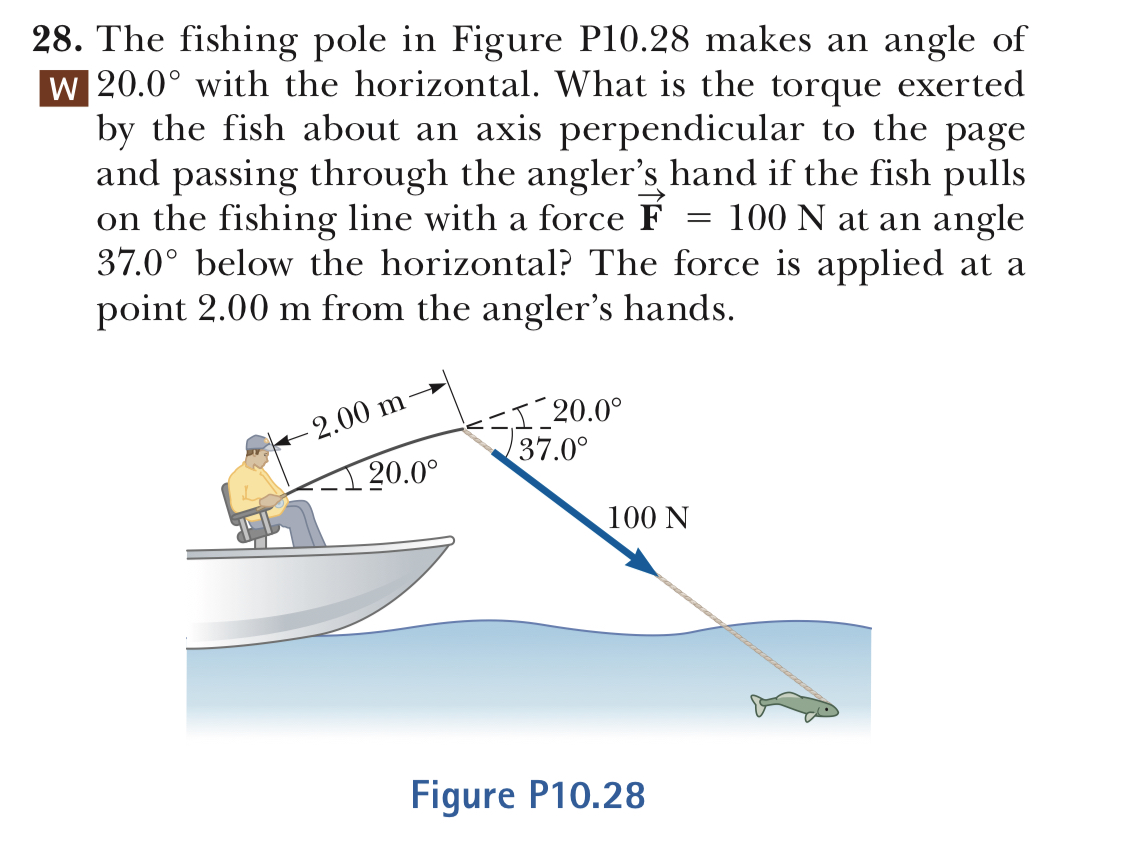28. The fishing pole in Figure P10.28 makes an angle ofW 20.0° with the horizontal. What is the torque exertedby the fish about an axis perpendicular to the pageand passing through the angler's hand if the fish pullson the fishing line with a force F - 100 N at an angle37.0° below the horizontal? The force is applied at apoint 2.00 m from the angler's hands.00 m37.020.0°100 NFigure P10.28

Questionhelp_outlineImage Transcriptionclose28. The fishing pole in Figure P10.28 makes an angle of W 20.0° with the horizontal. What is the torque exerted by the fish about an axis perpendicular to the page and passing through the angler's hand if the fish pulls on the fishing line with a force F - 100 N at an angle 37.0° below the horizontal? The force is applied at a point 2.00 m from the angler's hands. 00 m 37.0 20.0° 100 N Figure P10.28 fullscreen
Step 1

Given:  Force, F = 100 N

Radius, r = 2 m

Find: The torque exerted by the fish about an axis perpendicular to the page

Step 2

The angle will be 37o + 20o = 57o

Step 3

Use the equation of...

Want to see the full answer?

See Solution

Want to see this answer and more?

Our solutions are written by experts, many with advanced degrees, and available 24/7

See Solution
Tagged in

Physics